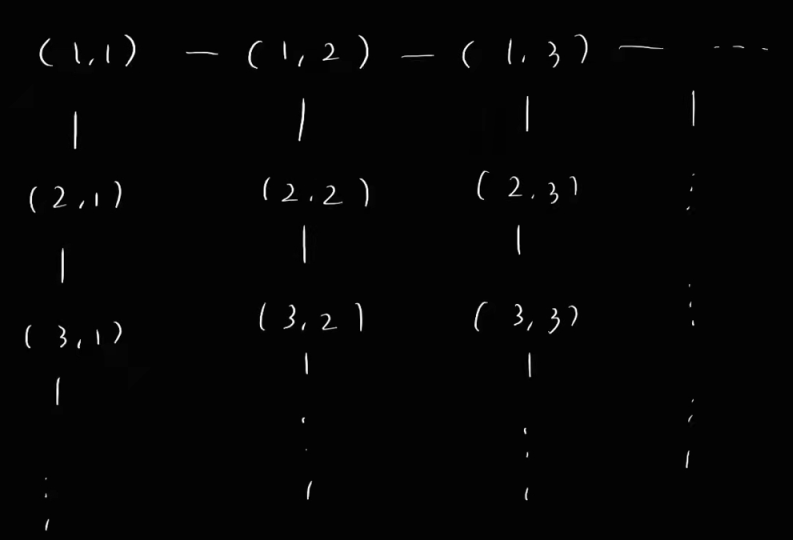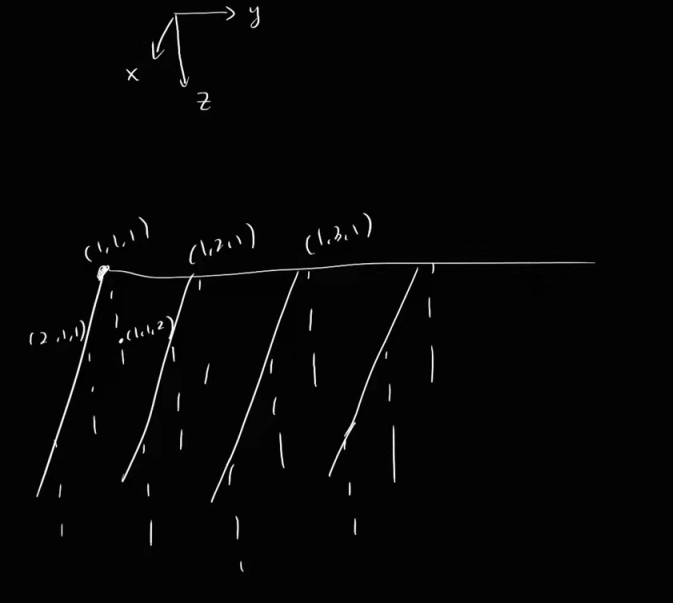• CST2021F 3-1 Circuit
• CST2021F 3-3 kth
• CST2021F 3-5 Prefix
• CST2021F 3-7 Sort

Problem White Box (20%) Black Box (80%)
CST2021F 3-1 Circuit (25%) 100  -1
CST2021F 3-3 kth (25%) 100  -1
CST2021F 3-5 Prefix (25%) 100  -1
CST2021F 3-7 Sort (25%) 100  -1

# CST2021F 3-1 Circuit

[关键词] Trie 树

## 方案设计

$\forall i \in [0, n)$，需要一种支持以下操作的数据结构：

(1) 持有 $[\max(i-k-1, 0), \min(i+k+1, n-1)]$ 中所有的元件的输出信息；

(2) 可以查询这些信息中满足与 $data[i]$ 异或值最大的 $data[j]$ 的 $j$，其中 $i \ne j$。

(3) 在考虑 $i$ 结束，转向考虑 $i+1$ 的时候，可以快速地删除 $data[i-k-1]$ 的信息，加入 $data[(i+1)+k+1]$ 的信息。

### 字典树：初始化

$\Sigma = \{0,1\}$​​ 的字典树的非叶结点只需记录其经过 $0$ 转移和 $1$ 转移后的到达结点，这里我们使用二叉树的记号，分别记作其左孩子和右孩子。

## 过程记录

(1) 撰写了对拍器 check.py（见附 1），但是本地的数据怎么测都是 AC。

(2) 撰写了 Trivial.cpp（见附 2），读入部分没变（事实上，最初我认为是后续字典树处理写出了问题，于是想写个最简单的版本交上去看下测试数据的规模），使用最平凡的 $O(nk)$ 的算法处理问题，最终发现还是爆零。目光转向读入部分，改用 scanf 后 Trivial 算法拿到了 40/50（自然会喜提 TLE），然后是将新的读入复制回 main.cpp，前后两次拿到了 50/50, 90/90, 完结撒花

## 复杂度分析

• 非叶结点，24M * 12Byte = 288M.
• 列表条目，0.5M * 12Byte = 6M.
• 叶结点，0.5M * 20Byte = 10M.
• $64$ 位整数的数据，0.5M * 8Byte = 4M.
• 合计 308M < 512M.

## 附录

### 附 1: check.py

import io
import time
import datetime
import random
import subprocess
import numpy as np

IN = ''
OUT = ''

class Judger:
def generateInputData(self):
global IN, OUT
IN = ''
OUT = ''
n = 10
k = 2
IN = f'{n} {k}\n'

dataList = [random.randint(0, 2**64-1) for i in range(n)]
rawList = [bin(data)[2:].zfill(64) for data in dataList]
print(dataList)
print(rawList)
for raw in rawList:
IN += f'{raw}\n'

for index, data in enumerate(dataList):
left = index - k - 1
if left < 0:
left = 0
right = index + k + 1
if right > n-1:
right = n-1

currMaxIndex = -999
currMaxValue = -999
for i in range(left, right+1):
if (i == index):
continue
if (dataList[i] ^ data) > currMaxValue:
currMaxIndex = i
currMaxValue = (dataList[i] ^ data)
OUT += f'{currMaxIndex}\n'

return IN

global OUT
return OUT.strip()

def judge(self, name, judgeAns=True):
print('\n\nCompiling...')
try:
subprocess.check_output(['g++', '-O2', '-std=c++14', '-w', f'{name}', '-o', 'main'])
except subprocess.CalledProcessError as e:
return 'CE'

print('Generating testing data...')
data = self.generateInputData()

print('Running...')
try:
time1 = time.time()
cppout = subprocess.check_output(['./main'], input=bytes(data, 'utf-8'), timeout=2.0)
cppout = cppout.decode('utf-8').strip()
time2 = time.time()
except subprocess.TimeoutExpired:
f = open(f'check-{datetime.datetime.now().strftime("%Y-%m-%d-%H-%M-%S")}.log', 'w+', encoding='utf-8')
f.write(f"Time Limit Exceeded\n")
f.write("-------------IN--------------\n")
f.write(data + '\n')
f.close()
return f'Time Limit Exceeded (2.000s)'
except subprocess.CalledProcessError as e:
f = open(f'check-{datetime.datetime.now().strftime("%Y-%m-%d-%H-%M-%S")}.log', 'w+', encoding='utf-8')
f.write(f"Runtime Error (signal {e.returncode})\n")
f.write("-------------IN--------------\n")
f.write(data + '\n')
f.close()
return f'Runtime Error (Signal {e.returncode})'
if judgeAns:
# print(cppout)
# print("↑ cppout ↓ ans")
# print(ans)

print('Judging...')
try:
cppout_ = cppout.split('\n')
ans_ = ans.split('\n')
if (len(cppout_) != len(ans_)):
raise BaseException(-1)
for i in range(len(cppout_)):
if (cppout_[i].strip() != ans_[i].strip()):
raise BaseException(i)
except BaseException as e:
f = open(f'check-{datetime.datetime.now().strftime("%Y-%m-%d-%H-%M-%S")}.log', 'w+', encoding='utf-8')
f.write("-------------IN--------------\n")
f.write(data + '\n')
f.write("-------------CPPOUT--------------\n")
f.write(cppout + '\n')
f.write("-------------ANS--------------\n")
f.write(ans + '\n')
f.close()
return f'Accepted ({round(time2 - time1, 3)}s)'

else:
return f'Program exited successfully ({round(time2 - time1, 4)}s)'

j = Judger()
for i in range(1000):
print(j.judge('trivial.cpp', judgeAns=True))


### 附 2: Trivial.cpp

#define ull unsigned long long
#include <cstdio>
int n, k;

ull data = {0};

int main() {
scanf("%d %d\n", &n, &k);
for (int i = 1; i <= n; ++i) {
char c = getchar();
while (c == '0' || c == '1') {
data[i] <<= 1;
data[i] += c - '0';
c = getchar();
}
}

for(int i = 1; i <= n; ++i) {

int l = i-k-1;
if(l < 1) l = 1;
int r = i + k + 1;
if(r > n) r = n;

ull currMax = 0;
ull currMaxIndex = l != i ? l : (l + 1);

for(int T = l; T <= r; ++T) {
if(T == i) {continue;}
if ((data[T]^data[i])>currMax) {
currMax = data[T] ^ data[i];
currMaxIndex = T;
}
}
printf("%d", currMaxIndex-1);
if(i != n) puts("");
}

return 0;
}

# CST2021F 3-3 kth

[关键词] 堆，偏序关系，归并排序

## 方案设计

### 二维情况

• $(i_1,j_1) \le (i_2, j_2)$, if $i_1 \le i_2$ and $j_1 \le j_2$.### 三维情况## 过程记录

• 首先对原有的 X，Y，Z 数组进行归并排序，具体来说是找到上述的 $X$​

• 编写 Index 类模拟上述定义的索引 tuple。可以简单地通过重载运算符来实现两个 Index 之间的比较（调用交互库函数）。

• 注意按照上述拓扑关系建立 Index 之间的新偏序关系，以保证所有顶点能且仅能被推入堆中一次。

# CST2021F 3-5 Prefix

[关键词] KMP next[] 表，动态规划

## 方案设计

### 关键概念定义

• 对于长度为 $n$ 的串 $S$，记之为 $S[1…n]$​. (Index starts from 1)
• 记 $next[j]$​ 表示 $S[1…j]$​​ 中最大自匹配的真前缀和真后缀的串长，也就是说，与讲义中偏重 Pattern String 下一次移动到的位置不同，我们更加侧重的是这个长度值。举个例子：
i 0 1 2 3 4 5 6
raw[i] * a a b a a b
next[i] -1 0 1 0 1 2 3

### next[] 表的构建

• 如果 $S[j+1]$​​ 与 $S[t+1]$​​ 相等，我们便找到了 $S[1…j+1]$​​ 的一个最大的自匹配的真前缀与真后缀，此即 $next[j+1] \leftarrow t+1$.
• 不然，我们转而考虑这个序列的下一个元素。

### 动态规划求前缀出现次数

i 0 1 2 3 4 5 6 7 8 9
raw[i] * a a b a a b a a b
next[i] -1 0 1 0 1 2 3 4 5 6
dp[i] - 0 1 0 1 2 1 2 3 2

## 过程记录

• 通过引入通配符 * 这个哨兵结点，可以有效地进行递推。
• 需要用 long long 来存储答案数值。
• 同时记录 $raw, next, dp$ 会导致内存溢出，因为 $(1+4+8)*20M = 260M > 256M.$
• 首先想到的解决方案是，由于答案的大小不会超过 $(20M)^2=4e14$，而 $\log_2(4*10^{14})=2+\frac {14} {\lg2} \approx 49$，使用 $56$ 个 bit 便可以将其储存。
• 于是我们可以撰写自己的 int56 类，在 get 与 set 对应整数的时候将 long long 通过位运算拆成 char, short, int 三部分（7 Byte）。
• 经过此种优化之后，内存用量为 $240M$，便有了通过的可能。
• 但是转而一想，数据结构并不是汇编程序设计。
• 事实上我们按照上述解题思路不难得出，我们是先算出了 $next$ 数组中的所有值，然后根据 $next$ 数组的值去递推 $dp$ 数组。
• 事实上，我们可以考虑只建立一个 $next$​ 数组，为 long long 类型，首先在其中算出所有 $next_j$，然后根据这个 $next_j$ 计算 $dp_i$，实行就地覆盖的方法，并不会影响答案的结果。

## 复杂度分析

KMP 的 next[] 表构建过程时间复杂度为 $O(n)$，之后根据 $next$ 递推 $dp$ 与累加求和的复杂度均为 $O(n)$。于是时间复杂度为 $O(n)$。

[关键词] 归并排序， 四路归并